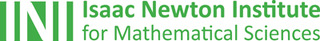# INI

## The Isaac Newton Institute: Creating eureka momentsOne of the most exciting places in the mathematical world is the Isaac Newton Institute for Mathematical Sciences (INI), an international research centre and our neighbour here on the University of Cambridge's maths campus.
The INI attracts leading mathematical scientists from all over the world, and is open to all. We are proud to be collaborating with the INI to bring the cutting edge mathematics that is being done there to the general public. The following content is part of this collaboration.

New perspectives in symmetry

What is group theory and why is it such an exciting area of maths? Two experts explain.

Maths in a minute: Cyclic groups

When things go round and round, a cyclic group may be just what you need!

Maths in a minute: Representing groups

Groups occur all over mathematics, so it makes sense to find a common language to talk about them all.

The magic of shuffling

Want to shuffle like a professional magician? Find out how to shuffle perfectly, imperfectly, and the magic behind it.

The mathematics of shuffling

A journey into the maths of card shuffling gives us a great insight into how mathematicians work.

• Want facts and want them fast? Our Maths in a minute series explores key mathematical concepts in just a few words.